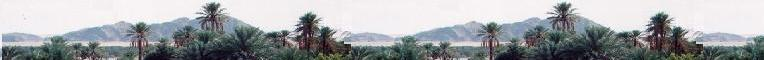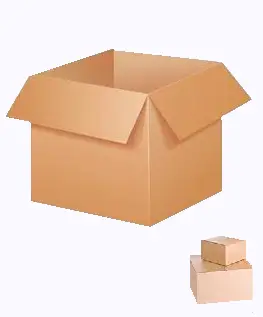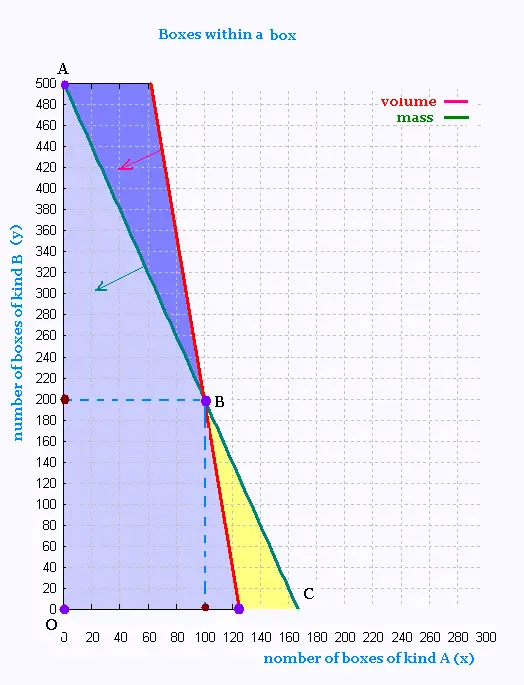# Mathematics 2: Linear optimization Examples

### BoxesA large box of volume 1 m3, and maximum weight of 50 kg, can contain two kinds of boxes:

Kind A: box of volume 8 dm3 which weights 300 g and costs 4 $, and Kind B: box of volume 1 dm3 which weights 100 g and costs 1$ .

Wow many boxes of the two different kinds can contain the large box in order to maximize the pofit?

Solution

Set the unknown:

x is the number of boxes of kind A
y is the number of boxes of kind B

Volume : 8 x + 1 y ≤ 1000
Mass : 300 x + 100 y ≤ 50 000

Or

Volume : 8 x + 1 y ≤ 1000
Mass : 3 x + y ≤ 500

Fonction objective: Z = 4 x + 1 y : maximum

We graph the following equations:

y = - 8 x + 1000
y = - 3 x + 500Vertex Z ($) O(0, 0) 0 A(0, 500) 500 B(100, 200) 600 C(1000/8, 0) = (125,0) 500 To obtain the maximum profit which is 600$, we would place within a large box, 100 boxes of kind A and 200 boxes of kind B.Web ScientificSentence

 chimie labs | scientific sentence | java | php | green cat | contact |